+1

# Overview of Artificial Neural Networks and its Applications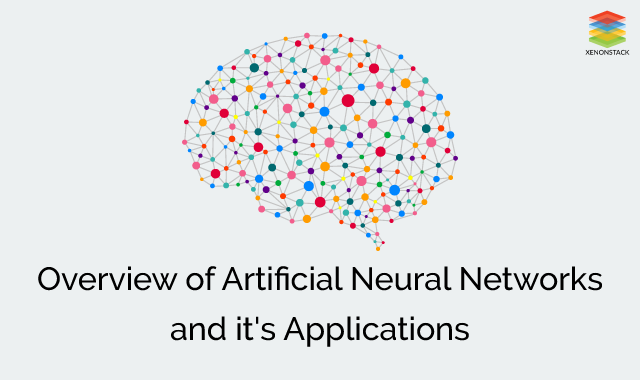## **What is Neural Network? **

The term ‘Neural’ is derived from the human (animal) nervous system’s basic functional unit ‘neuron’ or nerve cells which are present in the brain and other parts of the human (animal) body.

## Structure of Neurons in Brain****

The typical nerve cell of human brain comprises of four parts -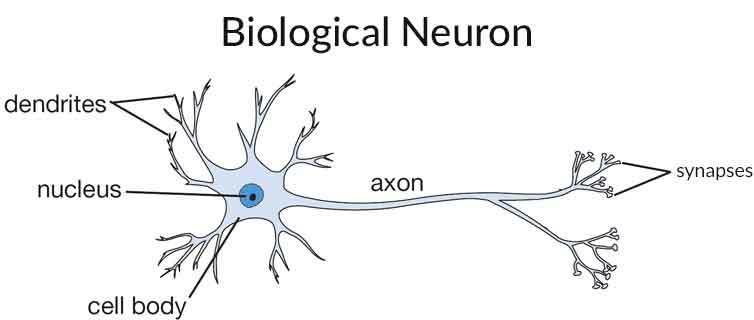Dendrite - It receives signals from other neurons.

Soma (cell body) - It sums all the incoming signals to generate input.

Axon - When the sum reaches a threshold value, neuron fires and the signal travels down the axon to the other neurons.

Synapses - The point of interconnection of one neuron with other neurons. The amount of signal transmitted depend upon the strength (synaptic weights) of the connections.

The connections can be inhibitory (decreasing strength) or excitatory (increasing strength) in nature.

So, neural network, in general, is a highly interconnected network of billions of neuron with trillion of interconnections between them.

## How is Brain Different from Computers?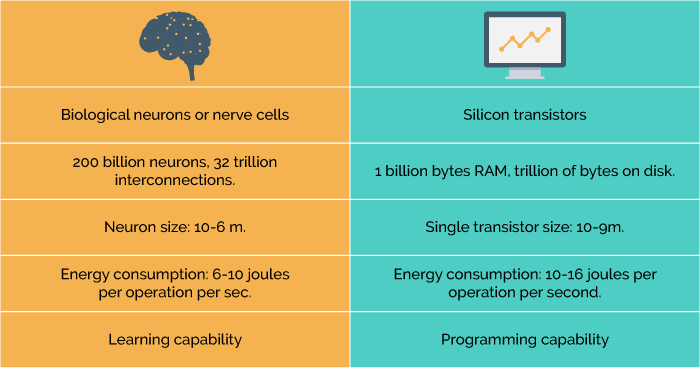## What is Artificial Neural Network?****

Artificial Neural Networks are the biologically inspired simulations performed on the computer to perform certain specific tasks like clustering, classification, pattern recognition etc.

Artificial Neural Networks, in general - is a biologically inspired network of artificial neurons configured to perform specific tasks.

## Similarity of ANN with Biological Neural Network

Neural networks resemble the human brain in the following two ways -

A neural network acquires knowledge through learning.

A neural network's knowledge is stored within inter-neuron connection strengths known as synaptic weights.

## Analogy of Artificial Neural Network With Biological Neural Network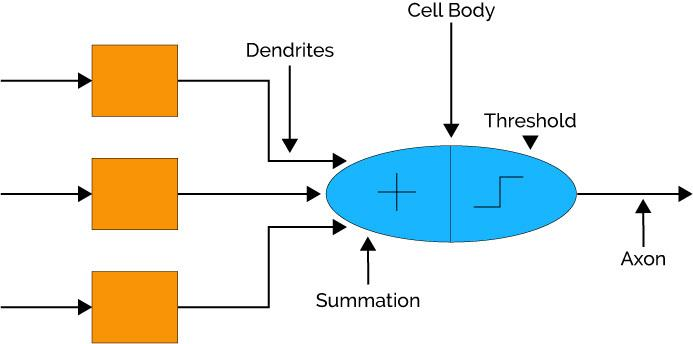The dendrites in biological neural network is analogous to the weighted inputs based on their synaptic interconnection in artificial neural network.

Cell body is analogous to the artificial neuron unit in artificial neural network which also comprises of summation and threshold unit.

Axon carry output that is analogous to the output unit in case of artificial neural network. So, ANN are modelled using the working of basic biological neurons.

## How Does Artificial Neural Network Works?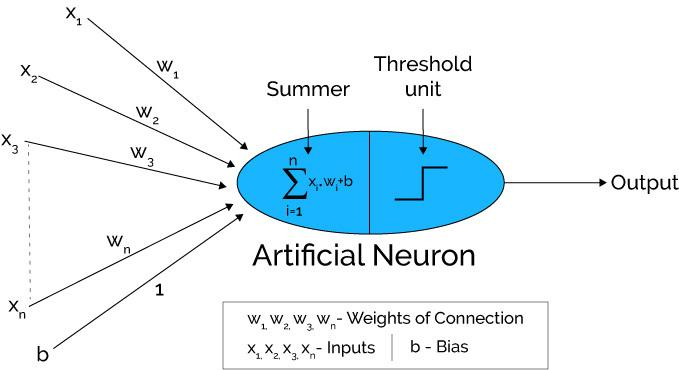Artificial neural networks can be viewed as weighted directed graphs in which artificial neurons are nodes and directed edges with weights are connections between neuron outputs and neuron inputs.

The Artificial Neural Network receives input from the external world in the form of pattern and image in vector form. These inputs are mathematically designated by the notation x(n) for n number of inputs.

Each input is multiplied by its corresponding weights. Weights are the information used by the neural network to solve a problem. Typically weight represents the strength of the interconnection between neurons inside the neural network.

The weighted inputs are all summed up inside computing unit (artificial neuron). In case the weighted sum is zero, bias is added to make the output not- zero or to scale up the system response. Bias has the weight and input always equal to ‘1'.

The sum corresponds to any numerical value ranging from 0 to infinity. In order to limit the response to arrive at desired value, the threshold value is set up. For this, the sum is passed through activation function.

The activation function is set of the transfer function used to get desired output. There are linear as well as the non-linear activation function.

Some of the commonly used activation function are - binary, sigmoidal (linear) and tan hyperbolic sigmoidal functions(nonlinear).

Binary - The output has only two values either 0 and 1. For this, the threshold value is set up. If the net weighted input is greater than 1, an output is assumed 1 otherwise zero.

Sigmoidal Hyperbolic - This function has ‘S’ shaped curve. Here tan hyperbolic function is used to approximate output from net input. The function is defined as - f (x) = (1/1+ exp(-𝝈x)) where 𝝈 - steepness parameter.

## Architecture of Artificial Neural Networks

A typical neural network contains a large number of artificial neurons called units arranged in a series of layers.

In typical artificial neural network, comprises different layers -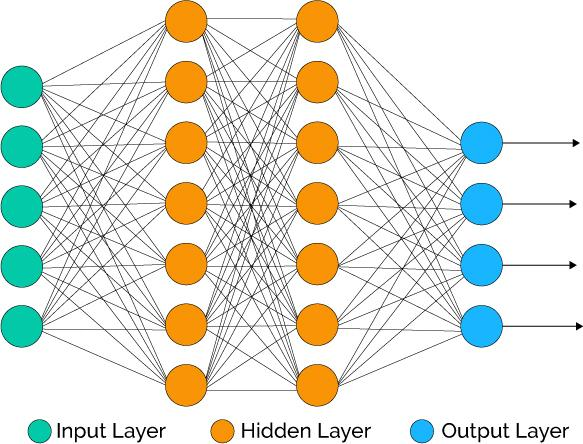Input layer - It contains those units (artificial neurons) which receive input from the outside world on which network will learn, recognize about or otherwise process.

Output layer - It contains units that respond to the information about how it's learned any task.

Hidden layer - These units are in between input and output layers. The job of hidden layer is to transform the input into something that output unit can use in some way.

Most neural networks are fully connected that means to say each hidden neuron is fully connected to the every neuron in its previous layer(input) and to the next layer (output) layer.

## Popular Neural Network Architectures

Perceptron - Neural Network having two input units and one output units with no hidden layers. These are also known as ‘single layer perceptrons.

Radial Basis Function Network - These networks are similar to the feed forward neural network except radial basis function is used as activation function of these neurons.

Multilayer Perceptron - These networks use more than one hidden layer of neurons, unlike single layer perceptron. These are also known as deep feedforward neural networks.

Recurrent Neural Network - Type of neural network in which hidden layer neurons has self-connections. Recurrent neural networks possess memory. At any instance, hidden layer neuron receives activation from the lower layer as well as it previous activation value.

Long /Short Term Memory Network (LSTM) - Type of neural network in which memory cell is incorporated inside hidden layer neurons is called LSTM network.

Hopfield Network - A fully interconnected network of neurons in which each neuron is connected to every other neuron. The network is trained with input pattern by setting a value of neurons to the desired pattern. Then its weights are computed. The weights are not changed. Once trained for one or more patterns, the network will converge to the learned patterns. It is different from other neural networks.

Boltzmann Machine Network - These networks are similar to Hopfield network except some neurons are input, while other are hidden in nature. The weights are initialized randomly and learn through back propagation algorithm.

Convolutional Neural Network - Get a complete overview of Convolutional Neural Networks through our blog Log Analytics with Machine Learning and Deep Learning.

## Other types of Neural Network

Modular Neural Network - It is the combined structure of different types of the neural network like multilayer perceptron, Hopfield network, recurrent neural network etc which are incorporated as a single module into the network to perform independent subtask of whole complete neural networks.

Physical Neural Network - In this type of artificial neural network, electrically adjustable resistance material is used to emulate the function of synapse instead of software simulations performed in the neural network.